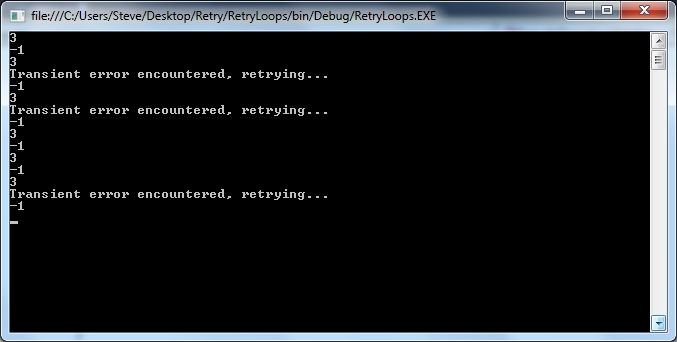Generic retry loops

Now more than ever I’m finding that the systems I write are required to communicate with a whole host of other systems. With “cloud computing” becoming ever more popular this trend will no doubt continue. Two obvious examples are databases and web-services (either directly or through APIs). Systems that rely on other applications and end-points naturally have extra failure points that we need to consider. More often than not, when calls to external end-points fail the problems are transient. That is to say if we try the same operation a little later the call magically works. There are many reasons why we experience transient errors, with some examples being insufficient band-width, transmission errors or servers being too busy to respond in a timely fashion. Of course, the end-point could be permanently down so our code should handle this gracefully, but usually pausing and retrying usually solves the problem.

Implementing a retry loop isn’t a difficult task, but given a class that calls a number of different methods of the same remote end-point, we don’t want to duplicate the retry code for each call. Fortunately, a relatively new feature in .NET makes implementing generic retry loops much easier. Using our old friend the console application (and with the same disclaimer about client profiles as always) I’ve written a calculator class that we will be calling using a retry loop:

using System;

namespace RetryLoops
{
public class Calculator
{

public Calculator()
{
unchecked
{
random = new Random((int)DateTime.Now.Ticks);
}
}

public int Add(int x, int y)
{
var value = random.Next(0, 5);

if (value == 0)
{
throw new InvalidOperationException("Transient error!");
}

return x + y;
}

public int Subtract(int x, int y)
{
var value = random.Next(0, 5);

if (value == 0)
{
throw new InvalidOperationException("Transient error!");
}

return x - y;
}
}
}

Note that the Add and Subtract methods have a 1 in 5 chance of throwing an exception when they’re called. This is to simulate transient errors. Note also that I’m using the seeding technique for random numbers that I introduced in my last post.

I’ve coded the Program class as follows:

using System;

namespace RetryLoops
{
public static class Program
{
public static void Main()
{
var calculator = new Calculator();

Console.WriteLine(calculator.Subtract(1, 2));
Console.WriteLine(calculator.Subtract(1, 2));
Console.WriteLine(calculator.Subtract(1, 2));
Console.WriteLine(calculator.Subtract(1, 2));
Console.WriteLine(calculator.Subtract(1, 2));
Console.WriteLine(calculator.Subtract(1, 2));

}
}
}

You don’t need to run this very many times before you get an unhandled exception.

Adding a method called ExecuteOperationWithRetry to the program and changing the main method to use this gives:

using System;

namespace RetryLoops
{
public static class Program
{
public static void Main()
{
var calculator = new Calculator();

Console.WriteLine(ExecuteOperationWithRetry(() => calculator.Subtract(1, 2)));
Console.WriteLine(ExecuteOperationWithRetry(() => calculator.Subtract(1, 2)));
Console.WriteLine(ExecuteOperationWithRetry(() => calculator.Subtract(1, 2)));
Console.WriteLine(ExecuteOperationWithRetry(() => calculator.Subtract(1, 2)));
Console.WriteLine(ExecuteOperationWithRetry(() => calculator.Subtract(1, 2)));
Console.WriteLine(ExecuteOperationWithRetry(() => calculator.Subtract(1, 2)));

}

private static TReturn ExecuteOperationWithRetry<TReturn>(Func<TReturn> operation)
{
const int MaximumAttempts = 10;
var timeBetweenRetries = TimeSpan.FromMilliseconds(100);

var attempts = 0;

while (true)
{
try
{
attempts++;
return operation.Invoke();
}
catch (Exception exception)
{
// If the exception isn't transient then re-throw:
if (!(exception is InvalidOperationException))
{
throw;
}

if (attempts >= MaximumAttempts)
{
throw;
}

// Wait before making another attempt:
Console.WriteLine("Transient error encountered, retrying...");
Thread.Sleep((int)(timeBetweenRetries.TotalMilliseconds * Math.Pow(2, attempts - 1)));
}
}
}
}
}

As the operation parameter of ExecuteOperationWithRetry is of type Func we can execute and retry any function we like without caring about the specific implementation of the function. In your own implementation you’ll probably want to get MaximumAttempts and timeBetweenRetries from a configuration file rather than hard-coding them, and you’ll probably also want to alter the exceptions that you retry on to suit the type of end-point. The Console.WriteLine statement in ExecuteOperationWithRetry is included only for demonstration purposes, and note that we are using the Math.Pow function to ensure that we wait a little longer each time we hit a transient error. This means that on the first attempt we wait for 100 milliseconds, then 200, 400, 800, 1600, etc… Once we’ve had 10 attempts we assume that the end-point is permanently broken and allow the exception to bubble up where (hopefully!) it’ll be caught by the calling method and handled appropriately.

When I ran this I got the following:…which proves the retry loop is doing its job.

To make the code even more generic we can add the following overload of the ExecuteOperationWithRetry method, enabling us to handle operations that don’t have return values:

private static void ExecuteOperationWithRetry(Action operation)
{
Func<bool> operationWithReturn = () =>
{
operation.Invoke();
return true;
};

ExecuteOperationWithRetry(operationWithReturn);
}

There is a lot of scope to improve this technique, and to tailor it to specific applications. In fact, it is possible to make a totally generic retry loop component that has the exceptions to retry, time to pause and number of attempts to make passed in as constructor arguments. I’ll cover this in a future article, but for now, this concludes my exploration of retry loops.